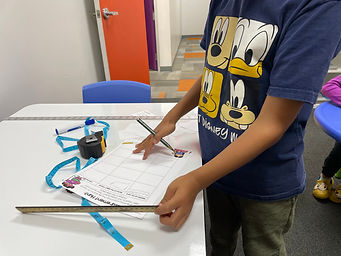## Mr. Jake

### Target 1​

###### Lesson Type:

New

Measurment

:

Comparison

Measure and compare objects in standard units.

###### 1:

Understand the units of measurement and their approximate measure.

###### 2:

Choose the appropriate unit based on knowledge of its measurement.

###### 3:

Understand approximately how small or large a measurement unit is.

###### 4:

Understand how to measure lengths with fractional increments.

5th

###### Vocabulary:

Inches, Centimeters, Feet, Yard, Meters, Unit of Measurement

Activities:

Students reviewed different units of measurement and identified which tools each unit of measurement could be found on.

Students drew ten cards with different lengths (i.e.: 18 inches, 1 meter, 5 centimeters) and found items around the building that equated to that measurement.### Home Exploration

###### Guiding Questions:## Absent Students:

Aaron

### Target 2

:

###### 1:

Study a given arithmetic sequence to determine what rule explains the sequence (i.e., 2-4-6-8-10…each number is the sequence gets bigger by 2).

###### 3:

Define multiplication and division patterns.

###### 4:

Understand that a pattern can be described by stating the rule that it is following.

###### 5:

Discover the arithmetic pattern present in Fibonacci’s Sequence.

5th

###### Vocabulary:

Sequence, Pattern

Activities:

Students matched sequences to their corresponding cards. Cards were either matched with arithmethc rules or fill-in-the-blanks that finished the sequence.### Home Exploration

###### Guiding Questions:### Target 3

:

###### Vocabulary:

Activities:### Home Exploration